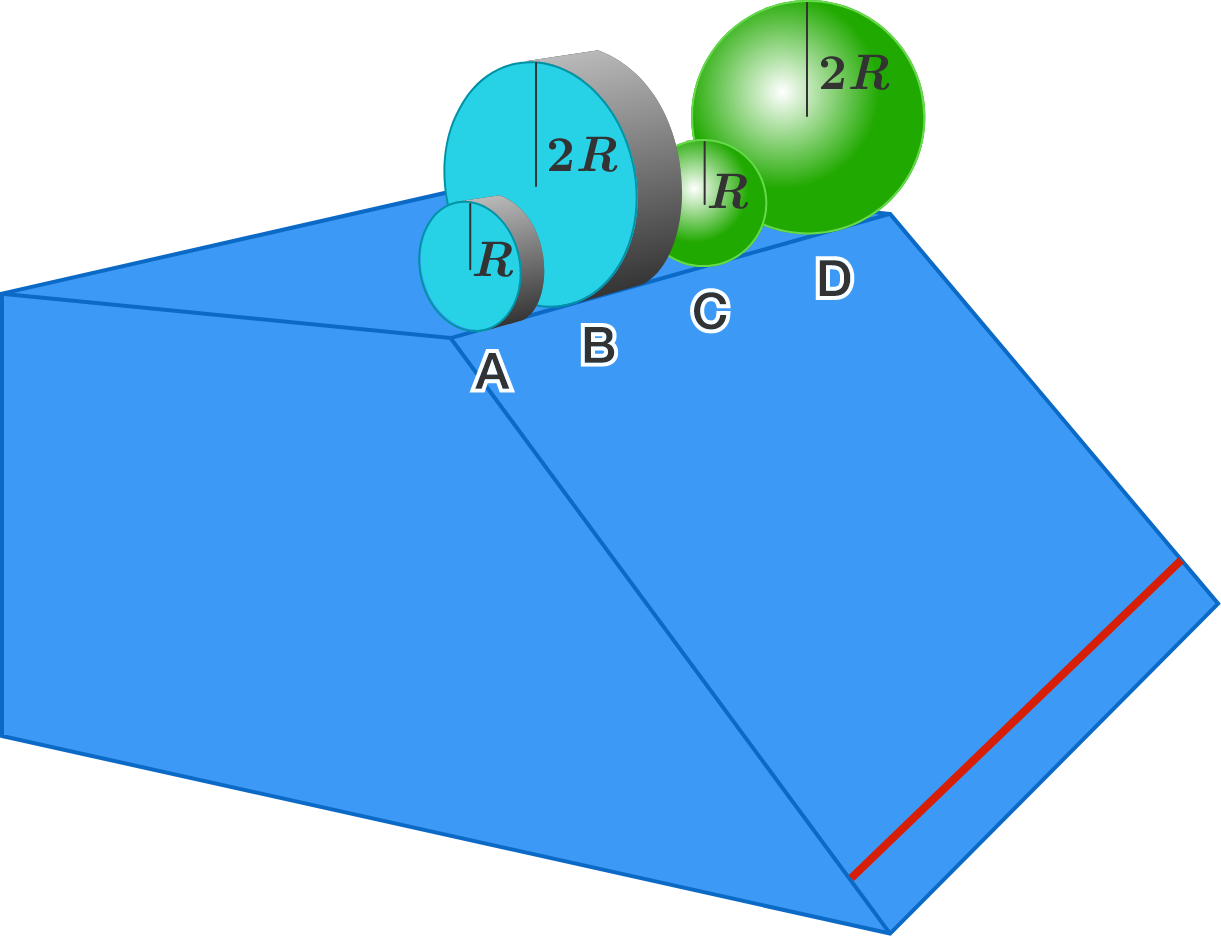# The great downhill race4 objects $A, B, C, D$ are made up of the same material. They are released, simultaneously from rest, from the starting line on the top of a rough inclined plane, so that they roll without slipping. $A$ and $B$ are cylinders, and $C$ and $D$ are spheres.

What is the order relation of the times taken by the objects to cross the finish line?
(A, B, C, D in the answer options denote the times taken by corresponding objects.)


Details and Assumptions:

• The objects are released with all their centers above the same starting line, which is the top edge of the ramp. They are considered to have crossed the finish line--the red line near the bottom edge--only if their centers have crossed the line when viewed from the side.

• Moment of inertia of a cylinder is $\frac{1}{2} MR^2$ and that of a sphere is $\frac{2}{5} MR^2$, where $M$ is the mass and $R$ is the radius of the respective objects.

• $B$ has twice the radius of $A,$ and $D$ has twice the radius of $C$.

×